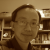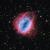# Platonic solid## Another Tetrahedron

Thanks to Knoblauch who created the Tetrahedron blog in VRMath2 Community. She demonstrated two methods to create a tetrahedron. Here I present another way to construct a tetrahedron using VRMath2 Editor. This is a generic way utilising the dihedral angles in 3D shapes. By this, many of the classical geometric solids such as those listed in this challenge can be easily created.## Dodecahedron

Dodecahedron is one of the five Platonic Solids. It consists of

• 12 faces (congruent pentagons)
• 30 edges
• 20 vertices (the meeting of 3 faces)## Octahedron

Octahedron is the third platonic solid. It consists of 8 faces (each is an equilateral triangle) and 6 vertices (each at the meeting of 4 faces).## Tetrahedron

The tetrahedron has the smallest number of faces in the five Platonic Solids, having only 4 faces. And in fact, four faces are the minimium requirement for a polyhedron. Other features of tetrahedron includes:

• Each face is an equilaterial triangle
• Each vertex is the meeting of 3 faces# Introduction

Platonic solids are polyhedron which satisfy 3 conditions

1. all its faces are congruent convex regular polygons,
2. none of its faces intersect except at their edges, and
3. the same number of faces meet at each of its vertices

## Hexahedron

The most commonly recognised platonic solid, also known as a cube.## My first Icosahedron

Icosahedron is the last of the five Platonic solids. It consists of 20 regular triangular faces.

I am sure that there is a more efficient way to do this...